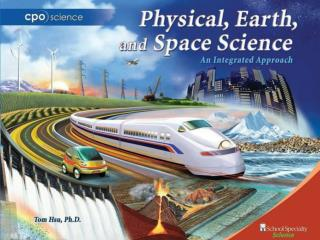DownloadDownload PresentationUNIT THREE: Matter, Energy, and Earth

# UNIT THREE: Matter, Energy, and Earth

Download Presentation## UNIT THREE: Matter, Energy, and Earth

- - - - - - - - - - - - - - - - - - - - - - - - - - - E N D - - - - - - - - - - - - - - - - - - - - - - - - - - -
##### Presentation Transcript

1. UNIT THREE: Matter, Energy, and Earth • Chapter 8 Matter and Temperature • Chapter 9 Heat • Chapter 10 Properties of Matter • Chapter 11 Earth’s Atmosphere and Weather

2. Chapter Eight: Matter and Temperature • 8.1 The Nature of Matter • 8.2 Temperature • 8.3 The Phases of Matter

3. Chapter 8.2 Learning Goals • Define temperature in terms of the motion of atoms and molecules. • Convert among Fahrenheit, Celsius, and Kelvin scales. • Describe the relationship between thermal energy and temperature.

4. 8.2 Temperature • There are two common temperature scales. • On the Fahrenheitscale, water freezes at 32 degrees and boils at 212 degrees. • The Celsius scale divides the interval between the freezing and boiling points of water into 100 degrees.

5. Solving Problems A friend in Paris sends you a recipe for a cake. The French recipe says to bake the cake at a temperature of 200 °C for 45 minutes. At what temperature should you set your oven, which uses the Fahrenheit scale?

6. Solving Problems • Looking for: • …temperature in degrees Fahrenheit • Given: • …temperature 200 C • Relationships: • TF = 9/5 TC + 32 • Solution • TF = (9/5)(200 °C) + 32 = 392 °F

7. 8.2 What temperature really is • Atoms are in constant motion, even in a solid object. • The back-and-forth jiggling of atoms is caused by thermal energy, which is a kind of kinetic energy.

8. 8.2 What temperature really is • Temperature measures the kinetic energy per molecule due to random motion.

9. 8.2 Thermometers • A thermometer is an instrument that measures the exact temperature. • Most thermometers contain either a silvery fluid (mercury) or a red fluid, which is alcohol containing a small amount of red dye.

10. 8.2 How a thermometer works • The volume of alcohol in a thermometer contains huge numbers of alcohol molecules. • As temperature increases, the alcohol molecules move faster and bounce off each other. • The liquid alcohol expands and takes up more space in the thermometer.

11. 8.2 Measuring temperature • A thermistoris a device that changes its electrical resistance as the temperature changes. • Some digital thermometers sense temperature by measuring the resistance of electrons passing through wire.

12. 8.2 Liquid-crystal thermometers • Some thermometers contain liquid crystals that change color based on temperature. • As temperature increases, the molecules of the liquid crystal bump into each other more and more. • This causes a change in the structure of the crystals, which in turn affects their color.

13. 8.2 Absolute zero • Absolute zero is -273°C. • You cannot have a temperature lower than absolute zero. • Think of absolute zero as the temperature at which atoms are “frozen.”

14. 8.2 Converting to Kelvin • The Kelvintemperature scale is useful in science because it starts at absolute zero. • To convert from Celsius to Kelvin, you add 273 to the temperature in Celsius.